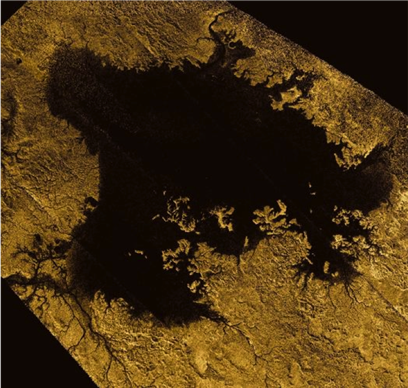Fluids: Liquids and Gases

Pressure in Liquids

Solve This! - An Extraterrestrial ProblemRadar mosaic of Legia Mare on Titan, about 350 x 420 km wide. NASA.

The P = ρgh equation means that pressure depends on depth within a liquid-filled container. So if the pressure at 10 m depth in a terrestrial lake is 9.8 x 104 Pascals, for 20 meters depth it is twice as much (19.6 x 104 Pa), and for 100 m is 10 times more (9.8 x 105 Pa).The increase in pressure with depth is dramatically illustrated by punching small holes at different depths in a tall, empty milk carton. When filled with water, the liquid coming out the bottom hole squirts further than water from higher holes because the pressure is greatest at the bottom.

Image: modified from © vectorlib.com - Fotolia.comSaturn’s moon Titan has lakes and seas of methane (CH4), which has a density of 421 kg/m3. Titan is smaller than Earth and is made of ice rather than rocks so its acceleration of gravity is only 1.35 m/s2. What is the pressure at 10 m below the surface of a sea on Titan?

Pa

P = ρgh = 421 kg/m3 * 1.35 m/s2 * 10 m

P = 5,684 Pa

How does that compare with pressure on Earth in the previous example?